# Иностранная литература и современность: General Statistics: Ch 6 HW Flashcards

Set Details Share
created 9 years ago by GreenHero64
155,145 views
This course is designed to acquaint the student with the principles of descriptive and inferential statistics. Topics will include: types of data, frequency distributions and histograms, measures of central tendency, measures of variation, probability, probability distributions including binomial, normal probability and student's t distributions, standard scores, confidence intervals, hypothesis testing, correlation, and linear regression analysis. This course is open to any student interested in general statistics and it will include applications pertaining to students majoring in athletic training, pre-nursing and business.
updated 9 years ago by GreenHero64
College: First year, College: Second year, College: Third year, College: Fourth year
Subjects:
statistics, mathematics, probability & statistics
Page to share:
Embed this setcancel
COPY
code changes based on your size selection
Size:
X

1

What requirements are necessary for a normal probability distribution to be a standard normal probability​ distribution?

The mean and standard deviation have the values of µ = 0 and σ = 1.

2

The waiting times between a subway departure schedule and the arrival of a passenger are uniformly distributed between 0 and 8 minutes. Find the probability that a randomly selected passenger has a waiting time less than 0.75 minutes.

0.098

​P(less than 0.75​) = (length of shaded ​region) x ​(height of shaded​ region)

= (0.75 – 0​) x ​(1 ÷ 8​) *round to 2 dec. places

=​(0.75​) x (0.13​) = 0.0975

3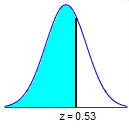Find the area of the shaded region. The graph depicts the standard normal distribution with mean 0 and standard deviation 1.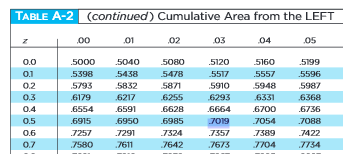The area of the shaded region is 0.7019.

4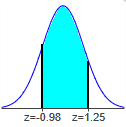Find the area of the shaded region. The graph depicts the standard normal distribution of bone density scores with mean 0 and standard deviation 1.

The area of the shaded region is 0.7309.

Area = (0.8944 – 0.1635)

5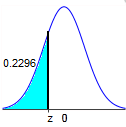Find the indicated z score. The graph depicts the standard normal distribution with mean 0 and standard deviation 1.

The indicated z score is -0.74.

6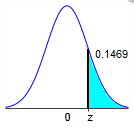Find the indicated z score. The graph depicts the standard normal distribution with mean 0 and standard deviation 1.

The indicated z score is 1.05.

Symmetric to Area of 0.1469 (z score of -1.05)

OR

Area = 1 – 0.1469

7

Assume that a randomly selected subject is given a bone density test. Those test scores are normally distributed with a mean of 0 and a standard deviation of 1.

Find the probability that a given score is less than 1.66 and draw a sketch of the region.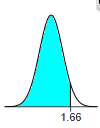The probability is 0.9515.

8

Assume the readings on thermometers are normally distributed with a mean of 0°C and a standard deviation of 1.00°C.

Find the probability that a randomly selected thermometer reads greater than –1.78 and draw a sketch of the region.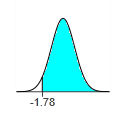The probability is 0.9625.

Symmetric to z-score of 1.78 (Area = 0.9625)

OR

1 – 0.0375

9

Assume the readings on thermometers are normally distributed with a mean of 0°C and a standard deviation of 1.00°C.

Find the probability that a randomly selected thermometer reads between –1.03 and –0.02 and draw a sketch of the region.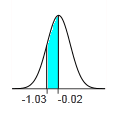The probability is 0.3405.

Area = (0.4920 – 0.1515)

10

Assume that a randomly selected subject is given a bone density test. Those test scores are normally distributed with a mean of 0 and a standard deviation of 1.

Find the probability that a given score is between –2.02 and 3.84 and draw a sketch of the region.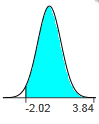The probability is 0.9782.

* For values of z above 3.48, use 0.9999

Area = (0.9999 – 0.0217)

11

Assume that the readings on the thermometers are normally distributed with a mean of 0° and standard deviation of 1.00°C. A thermometer is randomly selected and tested.

Draw a sketch and find the temperature reading corresponding to P97​, the 97th percentile. This is the temperature reading separating the bottom 97% from the top 3%.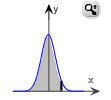The temperature for P97​ is approximately 1.88 °.

Area of 0.9700 ≈ 0.9699 (z score of 1.88)

12

Assume that the readings on the thermometers are normally distributed with a mean of and standard deviation of 1.00°C. Assume 2.1​% of the thermometers are rejected because they have readings that are too high and another 2.1​% are rejected because they have readings that are too low.

Draw a sketch and find the two readings that are cutoff values separating the rejected thermometers from the others.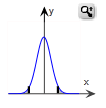The cutoff values are –2.03, 2.03 degrees.

Area values nearest 0.021 and 0.979

≈ 0.0212 and 0.9788

13

Find the indicated area under the curve of the standard normal​ distribution, then convert it to a percentage and fill in the blank.

About 72.86​% of the area is between z = –1.1 and z = 1.1 ​(or within 1.1 standard deviations of the​ mean).

14

Which of the following groups of terms can be used interchangeably when working with normal​ distributions?

areas, probability, and relative frequencies

​Area, probability,​ percentages, and relative frequencies are all used interchangeably when working with normal distributions.

15

Which of the following is NOT a requirement for a density​ curve?

The graph is centered around 0.

​"The graph is centered around​ 0" is not a requirement for a density curve.

16

Which of the following does NOT describe the standard normal​ distribution?

The graph is uniform.

The standard normal distribution is not uniform because its graph is​ bell-shaped.

17

Which of the following would be information in a question asking you to find the area of a region under the standard normal curve as a​ solution?

A distance on the horizontal axis is given

When given a​ z-score, you are usually finding the area of the shaded region under the standard normal curve. For the standard normal​ curve, a​ z-score is a distance along the horizontal axis.

18

Assume that a randomly selected subject is given a bone density test. Those test scores are normally distributed with a mean of 0 and a standard deviation of 1.

Find the probability that a given score is less than 3.61 and draw a sketch of the region.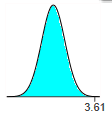The probability is 0.9999.

19

Assume that a randomly selected subject is given a bone density test. Bone density test scores are normally distributed with a mean of 0 and a standard deviation of 1.

Draw a graph and find P3, the 3rd percentile. This is the bone density score separating the bottom 3% from the top 97%.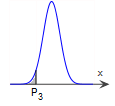The bone density score corresponding to Upper P 3 is –1.88.

Area = 0.0300 ≈ 0.0301

20

Find the indicated critical value.

z0.04

z0.04 = 1.75

Area = 0.0400 ≈ 0.0401

21

A survey found that​ women's heights are normally distributed with mean 63.6 in and standard deviation 2.3 in. A branch of the military requires​ women's heights to be between 58 in and 80 in.

1. Find the percentage of women meeting the height requirement. Are many women being denied the opportunity to join this branch of the military because they are too short or too​ tall?
2. If this branch of the military changes the height requirements so that all women are eligible except the shortest​ 1% and the tallest​ 2%, what are the new height​ requirements?

a. The percentage of women who meet the height requirement is 99.25​%.

*(58 – 63.6) ÷ 2.3 = -2.43 (min)

*(80 – 63.6) ÷ 2.3 = 7.13 (max)

P(-2.43 < z < 7.13)

= (1.0000 – 0.0075)

= 0.9925

No, because only a small percentage of women are not allowed to join this branch of the military because of their height.

b. For the new height​ requirements, this branch of the military requires​ women's heights to be at least 58.2 in and at most 68.3 in.

*shortest 1% = 0.0100 ≈ 0.0099 (value of -2.33)

*tallest 2% = 0.9800 ≈ 0.9798 (value of 2.05)

*µ + (x × σ)

63.6 + (-2.33 × 2.3) = 58.241

63.6 + (2.05 × 2.3) = 68.315

22

Men's heights are normally distributed with mean 68.7 in and standard deviation of 2.8 in. ​Women's heights are normally distributed with mean 63.5 in and standard deviation of 2.5 in. The standard doorway height is 80 in.

1. What percentage of men are too tall to fit through a standard doorway without​ bending, and what percentage of women are too tall to fit through a standard doorway without​ bending?
2. If a statistician designs a house so that all of the doorways have heights that are sufficient for all men except the tallest​ 5%, what doorway height would be​ used?

a. The percentage of men who are too tall to fit through a standard door without bending is 0​%.

*(80 – 68.7) ÷ 2.8 = 4.035714286 ≈ 4.04

P(z > 4.04)

= 1 – P(z < 4.04)

= 1 – 0.9999

= 0.0001 = 0%

The percentage of women who are too tall to fit through a standard door without bending is 0​%.

*(80 – 63.5) ÷ 2.5 = 6.6

P(z > 6.60)

= 1 – P(z < 6.60)

= 1 – 0.9999

= 0.0001 = 0%

b. The statistician would design a house with doorway height 73.3 in.

*tallest 5% = 0.9500 ≈ 1.645

µ + (x × σ)

68.7 + (1.645 × 2.8) = 73.306

23

The lengths of pregnancies are normally distributed with a mean of 266 days and a standard deviation of 15 days.

1. Find the probability of a pregnancy lasting 307 days or longer.
2. If the length of pregnancy is in the lowest 2​%, then the baby is premature. Find the length that separates premature babies from those who are not premature.

a. The probability that a pregnancy will last 307 days or longer is 0.0032.

*(307 – 266) ÷ 15 = 2.7333333 (z score)

P(z > 2.73)

= 1 – P(z < 2.73)

= 1 – 0.9977

= 0.0032

b. Babies who are born on or before 235 days are considered premature.

*lowest 2% = 0.0200 ≈ 0.0202 = -2.05

µ + (x × σ)

266 + (-2.05 × 15) = 235.25

24

Assume that the Richter scale magnitudes of earthquakes are normally distributed with a mean of 1.087 and a standard deviation of 0.562.

1. Earthquakes with magnitudes less than 2.000 are considered​ "microearthquakes" that are not felt. What percentage of earthquakes fall into this​ category?
2. Earthquakes above 4.0 will cause indoor items to shake. What percentage of earthquakes fall into this​ category?
3. Find the 95th percentile.
4. Will all earthquakes above the 95th percentile cause indoor items to​ shake?

a. 94.74​%

*(2.00 – 1.087) ÷ 0.562 = 1.62455516

P(z < 1.62) = 0.9474

b. 0%

*(4.00 – 1.087) ÷ 0.562 = 5.183274021

P(z > 5.18)

= 1 – P(z < 5.18)

= 1 – 0.9999

= 0.0001

c. 2.011

*95th = 0.9500 = 1.645 (z score)

µ + (x × σ)

1.087 + (1.645 × 0.562) = 2.01149

d. No, because not all earthquakes above the 95th percentile have magnitudes above 4.0.

25

Chocolate chip cookies have a distribution that is approximately normal with a mean of 23.1 chocolate chips per cookie and a standard deviation of 2.6 chocolate chips per cookie.

Find P5 and P95.

How might those values be helpful to the producer of the chocolate chip​ cookies?

P5 = 18.8

*P5 = 0.0500 = -1.645 (z score)

µ + (x × σ)

23.1 + (-1.645 × 2.6) = 18.823

P95 = 27.4

*95th = 0.9500 = 1.645 (z score)

µ + (x × σ)

23.1 + (1.645 × 2.6) = 27.377

The values can be used to identify cookies with an unusually low or high number of chocolate​ chips, so those numbers can be used to monitor the production process to ensure that the numbers of chocolate chips stays within reasonable limits.

26

Pulse rates of women are normally distributed with a mean of 77.5 beats per minute and a standard deviation of 11.6 beats per minute.

What are the values of the mean and standard deviation after converting all pulse rates of women to z scores using z = (x – ​µ) ÷ σ?

The original pulse rates are measure with units of​ "beats per​ minute". What are the units of the corresponding z​ scores?

µ = 0

σ = 1

The z scores are numbers without units of measurement.

27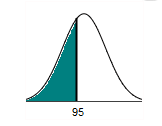Find the area of the shaded region. The graph to the right depicts IQ scores of​ adults, and those scores are normally distributed with a mean of 100 and a standard deviation of 15.

The area of the shaded region is 0.3707.

*z = (95 – 100) ÷ 15 = -0.333

P(z < -0.33) = 0.3707

28

Assume that adults have IQ scores that are normally distributed with a mean of µ = 100 and a standard deviation σ = 15.

Find the probability that a randomly selected adult has an IQ less than 118.

The probability that a randomly selected adult has an IQ less than 118 is 0.8849.

*z = (118 – 100) ÷ 15 = 1.2

P(z < 1.20) = 0.8849

29

Assume that adults have IQ scores that are normally distributed with a mean of 100 and a standard deviation 15. Find P3​, which is the IQ score separating the bottom 3​% from the top 97​%.

The IQ score that separates the bottom 10​% from the top 90​% is P3 = 71.8.

*bottom 3% = 0.0300 ≈ 0.0301 (z score = -1.88)

µ + (x × σ)

100 + (-1.88 × 15) = 71.8

30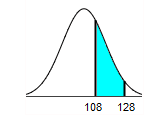Find the area of the shaded region. The graph to the right depicts IQ scores of​ adults, and those scores are normally distributed with a mean of 100 and a standard deviation of 15.

The area of the shaded region is 0.2674.

*z1 = (108 – 100) ÷ 15 = 0.533

*z2 = (128 – 100) ÷ 15 = 1.867

P(0.53 < z < 1.87)

= 0.9693 – 0.7019

= 0.2674

31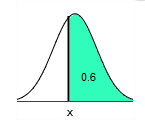Find the indicated IQ score. The graph to the right depicts IQ scores of​ adults, and those scores are normally distributed with a mean of 100 and a standard deviation of 15.

The indicated IQ​ score, x, is 96.3.

*1 – 0.6 = 0.4 ≈ 0.4013 (z score = -0.25)

µ + (x × σ)

= 100 + (-0.25 × 15)

= 96.25

32

Which of the following is not​ true?

1. A​ z-score is an area under the normal curve.
2. A​ z-score is a conversion that standardizes any value from a normal distribution to a standard normal distribution.
3. The area in any normal distribution bounded by some score x is the same as the area bounded by the equivalent​ z-score in the standard normal distribution.
4. If values are converted to standard​ z-scores, then procedures for working with all normal distributions are the same as those for the standard normal distribution.

A​ z-score is an area under the normal curve.

A​ z-score is not an area under the normal curve. Distances along the horizontal axis are represented by​ z-scores, while regions under the curve are represented by areas.

33

What conditions would produce a negative​ z-score?

a​ z-score corresponding to an area located entirely in the left side of the curve

A​ z-score corresponding to an area located entirely in the left side of the curve would produce a negative​ z-score.

34

Which of the following statistics are unbiased estimators of population​ parameters?

1. Sample proportion used to estimate a population proportion.
2. Sample range used to estimate a population range.
3. Sample variance used to estimate a population variance.
4. Sample mean used to estimate a population mean.
5. Sample standard deviation used to estimate a population standard deviation.
6. Sample median used to estimate a population median.

Sample proportion used to estimate a population proportion.

Sample variance used to estimate a population variance.

Sample mean used to estimate a population mean.

Sample​ means, sample​ proportions, and sample variances are unbiased estimators of population parameters. That​ is, their sampling distributions have a mean that is equal to the mean of the corresponding population parameter.

35

The assets​ (in billions of​ dollars) of the four wealthiest people in a particular country are 37, 27, 22, 19. Assume that samples of size n = 2 are randomly selected with replacement from this population of four values.

1. After identifying the 16 different possible samples and finding the mean of each​ sample, construct a table representing the sampling distribution of the sample mean. In the​ table, values of the sample mean that are the same have been combined.
2. Compare the mean of the population to the mean of the sampling distribution of the sample mean.
3. Do the sample means target the value of the population​ mean? In​ general, do sample means make good estimates of population​ means? Why or why​ not?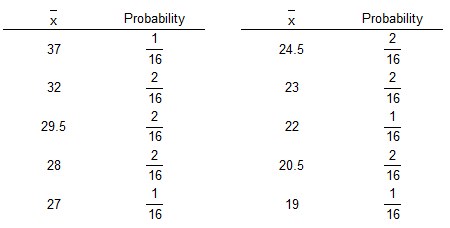a. (see picture)

37-37; 37-27; 37-22; 37-19; 27-37; 27-27; 27-22; 27-19; 22-37; 22-27; 22-22; 22-19; 19-37; 19-27; 19-22; 19-19 – (16 samples)

The distinct means are 37​, 32​, 29.5​, 28, 27, 24.5​, 23, 22​, 20.5, and 19. To find the corresponding probability for each​ mean, divide the number of occurrences of that mean by the total number of samples.

b. The mean of the​ population, 26.25​, is equal to the mean of the sample​ means, 26.25.

Recall that the mean of a set of data is the measure of center found by adding the data values and dividing the total by the number of data values.

(37, 27, 22, 19) ÷ 4 = 26.25

c. The sample means target the population mean. In​ general, sample means do make good estimates of population means because the mean is an unbiased estimator.

36

Which of the following is NOT a property of the sampling distribution of the sample​ mean?

1. The sample means target the value of the population mean.
2. The distribution of the sample mean tends to be skewed to the right or left.
3. The mean of the sample means is the population mean.
4. The expected value of the sample mean is equal to the population mean.

The distribution of the sample mean tends to be skewed to the right or left.

This is not a property of the sampling distribution. The distribution of the sample means tends to be a normal distribution.

37

Which of the following is a biased​ estimator? That​ is, which of the following does not target the population​ parameter?

Median

The median is a biased estimator. It does not target the population parameter.

38

The population of current statistics students has ages with mean µ and standard deviation σ. Samples of statistics students are randomly selected so that there are exactly 37 students in each sample. For each​ sample, the mean age is computed.

What does the central limit theorem tell us about the distribution of those mean​ ages?

Because n > 30, the sampling distribution of the mean ages can be approximated by a normal distribution with mean µ and standard deviation σ ÷ √(37) .

The central limit theory applies whenever n > 30. Because n > ​30, the sampling distribution of the mean ages can be approximated by a normal distribution with mean µ and standard deviation σ ÷ √(n) .

39

Which of the following is NOT a conclusion of the Central Limit​ Theorem?

1. The standard deviation of all sample means is the population standard deviation divided by the square root of the sample size.
2. The mean of all sample means is the population mean µ.
3. The distribution of the sample means ​will, as the sample size​ increases, approach a normal distribution.
4. The distribution of the sample data will approach a normal distribution as the sample size increases.

The distribution of the sample data will approach a normal distribution as the sample size increases.

The Central Limit Theorem applies to the sampling distribution of and not to the distribution of the sample data.

40

Which of the following is not a commonly used​ practice?

1. If the distribution of the sample means is normally​ distributed, and n > 30, then the population distribution is normally distributed.
2. The distribution of sample means gets closer to a normal distribution as the sample size n gets larger.
3. If the original population is not normally distributed and n > 30, the distribution of the sample means can be approximated reasonably well by a normal distribution.
4. If the original population is normally​ distributed, then for any sample size​ n, the sample means will be normally distributed.

If the distribution of the sample means is normally​ distributed, and n > 30, then the population distribution is normally distributed.

This is not always the case because skewed population distributions may have sampling distributions that are normally distributed.

41

Assume that​ women's heights are normally distributed with a mean given by µ = 63.6 in​, and a standard deviation given by σ = 2.6 in.

(a) If 1 woman is randomly​ selected, find the probability that her height is less than 64 in.

(b) If 44 women are randomly​ selected, find the probability that they have a mean height less than 64 in.

​(​a) The probability is approximately 0.5596.

(64 – 63.6) ÷ 2.6

= 0.1538461538

≈ 0.15

Area to the left of z = 0.15 is approximately 0.5596

(b) The probability is approximately 0.8461.

σ = σ ÷ √(n)

= 2.6 ÷ √(44)

≈ 0.391964748

z ≈ (64 – 63.6) ÷ 0.391964748

≈ 1.020499935

≈ 1.02

Area to the left of z = 1.02 is approximately 0.8461

42

Assume that​ women's heights are normally distributed with a mean given by µ = 62.2 in​, and a standard deviation given by σ = 2.8 in.

1. If 1 woman is randomly​ selected, find the probability that her height is between 62.0 in and 63.0 in.
2. If 15 women are randomly​ selected, find the probability that they have a mean height between 62.0 in and 63.0 in.

a. The probability is approximately 0.1420.

zlow = (62.0 – 62.2) ÷ 2.8

= -0.0714285714 ≈ -0.07

zup = (63.0 – 62.2) ÷ 2.8

= 0.2857142857 ≈ 0.29

The probability is the area between zlow = -0.07 and zup = 0.29 under the standard normal distribution.

Area = 0.6141 – 0.4721 = 0.1420

b. The probability is approximately 0.4768.

σ = σ ÷ √(n)

= 2.8 ÷ √(15)

≈ 0.7229568913

zlow = (62.0 – 62.2) ÷ 0.7229568913

≈ -0.2766416676

≈ -0.28

zup ≈ (63.0 – 62.2) ÷ 0.47229568913

≈ 1.10656667

≈ 1.11

The probability is the area between z = -0.28 and z = 1.11 under the standard normal distribution.

0.8665 – 0.3897 = 0.4768

43

Women have head circumferences that are normally distributed with a mean given by µ = 24.27 in​., and a standard deviation given by σ = 0.8 in.

1. If a hat company produces​ women's hats so that they fit head circumferences between 23.6 in. and 24.6 ​in., what is the probability that a randomly selected woman will be able to fit into one of these​ hats?
2. If the company wants to produce hats to fit all women except for those with the smallest 1.25​% and the largest 1.25​% head​ circumferences, what head circumferences should be​ accommodated?
3. If 12 women are randomly​ selected, what is the probability that their mean head circumference is between 23.6 in. and 24.6 ​in.?
4. If this probability is​ high, does it suggest that an order of 12 hats will very likely fit each of 12 randomly selected​ women? Why or why​ not? (Assume that the hat company produces​ women's hats so that they fit head circumferences between 23.6 in. and 24.6 ​in.)

a. The probability is 0.4586.

zlow = (23.6.0 – 24.27) ÷ 0.8

≈ -0.84

zup = (24.6 – 24.27) ÷ 0.8

≈ 0.41

The probability is the area between zlow = -0.84 and zup = 0.41 under the standard normal distribution.

Area = 0.6591 – 0.2005 = 0.4586

b. The minimum head circumference accommodated should be 22.48 in.

The maximum head circumference accommodated should be 26.06 in.

smallest 1.25% = 0.0125 --> z = -2.24

largest 1.25% = 0.9875 --> z = 2.24

min = (-2.24)(0.8) + 24.27 = 22.478

max = (2.24)(0.8) + 24.27 = 26.062

c. The probability is 0.9217.

σ = σ ÷ √(n)

= 0.8 ÷ √(12)

≈ 0.2309401077

zlow = (23.6 – 24.27) ÷ 0.2309401077

≈ -2.90

zup ≈ (24.6 – 24.27) ÷ 0.2309401077

≈ 1.43

The probability is the area between z = -2.90 and z = 1.43 under the standard normal distribution.

0.9236 – 0.0019 = 0.9217

d. No, the hats must fit individual​ women, not the mean from 12 women. If all hats are made to fit head circumferences between 23.6 in. and 24.6 ​in., the hats​ won't fit about half of those women.

44

A ski gondola carries skiers to the top of a mountain. It bears a plaque stating that the maximum capacity is 12 people or 1920 lb. That capacity will be exceeded if 12 people have weights with a mean greater than (1920 lb)/12 = 160 lb. Assume that weights of passengers are normally distributed with a mean of 177.5 lb and a standard deviation of 40.4 lb.

1. Find the probability that if an individual passenger is randomly​ selected, their weight will be greater than 160 lb.
2. Find the probability that 12 randomly selected passengers will have a mean weight that is greater than 160 lb​ (so that their total weight is greater than the gondola maximum capacity of 1920 ​lb)

a. 0.6664

z = (160 – 177.5) ÷ 40.4

= -0.43

The cumulative area is 0.3336

Subtract this probability from 1 to find the probability that the​ passenger's weight is greater than 160 lb.

1 – 0.3336 = 0.6664

b. 0.9332

z = (x̄ – µ) ÷ (σ / √(n) )

= (160 – 177.5) ÷ (40.4 / √(12) )

= -1.50

The cumulative area is 0.0668

Subtract this probability from 1 to find the probability that the​ sample mean is greater than 160 lb.

1 – 0.0668 = 0.9332

c. No, there is a high probability that the gondola will be overloaded if it is occupied by 12 ​passengers, so it appears that the number of allowed passengers should be reduced.

The gondola will be overloaded if the mean weight of the passengers is above the maximum allowed mean weight.

45

An engineer is going to redesign an ejection seat for an airplane. The seat was designed for pilots weighing between 150 lb and 191 lb. The new population of pilots has normally distributed weights with a mean of 156 lb and a standard deviation of 25.7 lb.

1. If a pilot is randomly​ selected, find the probability that his weight is between 150 lb and 191 lb.
2. If 33 different pilots are randomly​ selected, find the probability that their mean weight is between 150 lb and 191 lb.
3. When redesigning the ejection​ seat, which probability is more​ relevant?

a. The probability is approximately 0.5041.

zlow = (150 – 156) ÷ 25.7

≈ -0.23

zup = (191 – 156) ÷ 25.7

≈ 1.36

The probability is the area between zlow = -0.23 and zup = 1.36 under the standard normal distribution.

Area = 0.9131 – 0.4090 = 0.5041

b. The probability is approximately 0.9098.

σ = σ ÷ √(n)

= 25.7 ÷ √(33)

≈ 4.473795758

zlow = (150 – 156) ÷ 4.473795758

≈ -1.34

zup ≈ (191 – 156) ÷ 4.473795758

≈ 7.82

The probability is the area between z = -1.34 and z = 7.82 under the standard normal distribution.

0.9999 – 0.0901 = 0.9098

c. Part (a) because the seat performance for a single pilot is more important.

46

An airliner carries 200 passengers and has doors with a height of 72 in. Heights of men are normally distributed with a mean of 69.0 in and a standard deviation of 2.8 in.

1. If a male passenger is randomly​ selected, find the probability that he can fit through the doorway without bending.
2. If half of the 200 passengers are​ men, find the probability that the mean height of the 100 men is less than 72 in.
3. When considering the comfort and safety of​ passengers, which result is more​ relevant: the probability from part​ (a) or the probability from part​ (b)? Why?
4. When considering the comfort and safety of​ passengers, why are women ignored in this​ case?

a. The probability is 0.8577.

z = (72 – 69.0) ÷ 2.8

= 1.07

The cumulative area is 0.8577

b. The probability is 0.9999.

z = (x̄ – µ) ÷ (σ / √(n) )

= (72 – 69.0) ÷ (2.8 / √(100) )

= 10.71

The cumulative area is 0.9999

c. The probability from part​ (a) is more relevant because it shows the proportion of male passengers that will not need to bend.

d. Since men are generally taller than​ women, a design that accommodates a suitable proportion of men will necessarily accommodate a greater proportion of women.

47

Before every​ flight, the pilot must verify that the total weight of the load is less than the maximum allowable load for the aircraft. The aircraft can carry 35 ​passengers, and a flight has fuel and baggage that allows for a total passenger load of 5,880 lb. The pilot sees that the plane is full and all passengers are men. The aircraft will be overloaded if the mean weight of the passengers is greater than (5,880 lb)/35 = 168 lb.

What is the probability that the aircraft is​ overloaded?

Should the pilot take any action to correct for an overloaded​ aircraft? Assume that weights of men are normally distributed with a mean of 175.5 lb and a standard deviation of 38.7.

The probability is approximately 0.8749.

σ = σ ÷ √(n)

= 38.7 ÷ √(35)

≈ 6.541493932

z = (168 – 175.5) ÷ 6.541493932

≈ -1.15

The cumulative area is 0.1251

Subtract this area from 1 to find the area to the right.

1 – 0.1251 = 0.8749

Yes. Because the probability is​ high, the pilot should take action by somehow reducing the weight of the aircraft.

48

The value given below is discrete. Use the continuity correction and describe the region of the normal distribution that corresponds to the indicated probability.

Probability of exactly 5 passengers who do not show up for a flight

The area between 4.5 and 5.5

49

If np ≥ 5 and nq ≥ 5, estimate P(more than 5) with n = 12 and p = 0.4 by using the normal distribution as an approximation to the binomial​ distribution; if np < 5 or nq < 5, then state that the normal approximation is not suitable.

The normal distribution cannot be used.

np = (12)(0.4) = 4.8 < 5

50

If np ≥ 5 and nq ≥ 5, estimate P(more than 5) with n = 13 and p = 0.6 by using the normal distribution as an approximation to the binomial​ distribution; if np < 5 or nq < 5, then state that the normal approximation is not suitable.

P(at least 8) = 0.567

µ = np = (13)(0.6) = 7.8

σ = √(npq) = √(13×0.6×0.4) = 1.766352173

z = (x – 0.5 – µ) ÷ σ

= (8 – 0.5 – 7.8) ÷ 1.766352 = -0.17

Area = 0.4325

P(at least 8) = 1 – P(less than 8)

= 1 – 0.433

= 0.567

51

A scientist conducted a hybridization experiment using peas with green pods and yellow pods. He crossed peas in such a way that​ 25% (or 144​) of the 576 offspring peas were expected to have yellow pods. Instead of getting 144 peas with yellow​ pods, he obtained 149. Assume that the rate of​ 25% is correct.

1. Find the probability that among the 576 offspring​ peas, exactly 149 have yellow pods.
2. Find the probability that among the 576 offspring​ peas, at least 149 have yellow pods.
3. Which result is useful for determining whether the claimed rate of​ 25% is​ incorrect? (Part​ (a) or part​ (b)?)
4. Is there strong evidence to suggest that the rate of​ 25% is​ incorrect?

a. The probability that exactly 149 have yellow pods is 0.0355.

b. The probability that at least 149 have yellow pods is 0.3336.

c. Part (b) is useful for determining whether the claimed rate is incorrect.

d. No

52

A​ gender-selection technique is designed to increase the likelihood that a baby will be a girl. In the results of the​ gender-selection technique, 809 births consisted of 422 baby girls and 387 baby boys. In analyzing these​ results, assume that boys and girls are equally likely.

1. Find the probability of getting exactly 422 girls in 809 births.
2. Find the probability of getting 422 or more girls in 809 births. If boys and girls are equally​ likely, is 422 girls in 809 births unusually​ high?
3. Which probability is relevant for trying to determine whether the technique is​ effective: the result from part​ (a) or the result from part​ (b)?
4. Based on the​ results, does it appear that the​ gender-selection technique is​ effective?

a. The probability of getting exactly 422 girls in 809 births is 0.0131.

b. The probability of getting 422 or more girls in 809 births is 0.1151.

c. No, because 422 girls in 809 births is not far from what is​ expected, given the probability of having a girl or a boy.

d. No​, because the probability of having 422 or more girls in 809 births is not p​unlikely, and​ thus, is attributable to random chance.

53

In a survey of 1227 ​people, 828 people said they voted in a recent presidential election. Voting records show that 65​% of eligible voters actually did vote. Given that 65​% of eligible voters actually did​ vote.

1. Find the probability that among 1227 randomly selected​ voters, at least 828 actually did vote.
2. What do the results from part​ (a) suggest?

(a) P(X ≥ 828) = 0.0359

(b) Some people are being less than honest because ​P(x ≥ 828​) is less than​ 5%.

54

Based on a recent​ survey, 20​% of adults in a specific country smoke. In a survey of 100 ​students, it is found that 18 of them smoke.

Find the probability that should be used for determining whether the 20​% rate is correct for students.

What can be​ concluded?

The probability that should be used for determining whether the 20​% rate is correct is 0.352.

There is not very strong evidence against the 20​% rate because this probability is not very small.

55

Which of the following is NOT a requirement for using the normal distribution as an approximation to the binomial​ distribution?

1. The sample is a simple random sample of size n from a population in which the proportion of successes is​ p, or the sample is the result of conducting n independent trials of a binomial experiment in which the probability of success is p.
2. nq ≥ 5
3. The sample is the result of conducting several dependent trials of an experiment in which the probability of success is p.
4. np ≥ 5

The sample is the result of conducting several dependent trials of an experiment in which the probability of success is p.

Having the sample as the result of conducting several dependent trials of an experiment in which the probability of success is p is not a requirement for using the normal distribution as an approximation to the binomial distribution.

56

Which statement below indicates the area to the left of 19.5 before a continuity correction is​ used?

1. At least 19
2. At least 20
3. Less than 19
4. At most 19

At most 19

​"At most​ 19" indicates the area to the left of 19.5 before a continuity correction is used.

57

A continuity correction is made to a discrete whole number x in the binomial distribution by representing the discrete whole number x by which of the following​ intervals?

x – 0.5 to x + 0.5

The interval is x–0.5 to x+0.5 because 0.5 needs to be added and subtracted from x to create the interval.